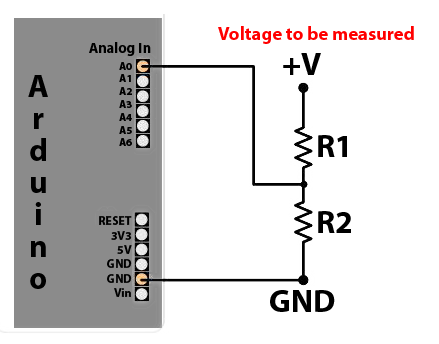#### Post### Calculating Sensor battery life

#### Theoretical Battery Life

The battery life (for a hypothetical temperature sensor) can be calculated by determining the average current for the circuit, using the following general equation:

Iavg = (t0I0 + t1I1 ... + tx*Ix) / (t0 + t1 ... + tx)

Note these figures are for illustration purposes - your results will certainly vary. Current is measured at the battery with a battery Voltage of 3.0V.

Idling: I0 = 28 mA, t0 = 0.65 sec (using the delays I set up in the sketch) Transmitting: I1 = 31 mA, t1 = say 50 mSec (time is just a guess) Sleeping: I2 = 120 uA (very roughly), t2 = 15 minutes (the temperature sampling rate)

Using the above figures the effective current = 0.142 mA. The sleep current is difficult to measure accurately, so this figure may vary and will affect the lifetime calculation.

Assume the batteries are good for 2000 mAhr, that gives a lifetime of 14,101 hours, which equals to 19.6 months. The lifetime is dominated by the sleep current and sensor sample rate. Assuming the figures and calculations are correct (let us know if they aren't), it's likely the batteries would die from old age rather than through actual usage.

The Arduino 3.3V 8mhz can handle down to roughly 2.8V and the Nrf24l01+ down to 1.9V.

To be able to suck as much juice out of the batteries as possible you can use a DC-DC Step up booster. This booster will convert everything from 0.8V back to 3.3V. Note that a booster can be a bit noisy (and disturb the radio).

It can help to connect a 0,1uF ceramic capacitor from GND to VOut. To avoid noise to the radio (NRF24l01+) you can power it directly from the batteries since it can operate down to 1.9V and only power the arduino through the booster.

#### Measuring and Reporting Battery LevelUse a 1MΩ (R1) and 470KΩ (R2) resistor in series, connected to the positive terminal on the battery and ground and then connected the tap point to the A0 input on the CPU.

The tap point could be bypassed with a 0.1 uF capacitor to keep the noise level low, at this otherwise high impedance point.

The ADC is set to use the internal reference value of 1.1V - so Vmax at ADCmax = 1.1*(16+4703)/4703 = 3.44V

The battery power value must be converted to a percentage compatable with your Controller.

Posted in Arduino, WSN 06 Mar 2017